# Cherries

Cherries in the bowl can be divided equally among 19 or 13 or 28 children. How many are the minimum cherries in the bowl?

n =  6916

### Step-by-step explanation:Did you find an error or inaccuracy? Feel free to write us. Thank you!Tips to related online calculators
Do you want to calculate least common multiple two or more numbers?
Do you solve Diofant problems and looking for a calculator of Diofant integer equations?

## Related math problems and questions:

• PlumsIn the bowl are plums. How many would be there if we can divide it equally among 8, 10 and 11 children?
• FractionsSort fractions z1 = (6)/(11); z2 = (10)/(21); z3 = (19)/(22) by its size. Result write as three serial numbers 1,2,3.
• GroupA group of kids wanted to ride. When the children were divided into groups of 3 children, one remain. When divided into groups of 4 children, 1 remains. When divided into groups of 6 children, 1 remains. After divided into groups of 5 children, no one lef
• The smallest numberWhat is the smallest number that can be divided by both 5 and 7
• Nuts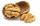How many we must have at least nuts if we can equally divide it to 10 children, 12 children or 15 children and any nut left?
• Apples 2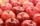How many minimum apples are in the cart, if possible is completely divided into packages of 6, 14 and 21 apples?
• LCMCommon multiple of three numbers is 3276. One number is in this number 63 times, second 7 times, third 9 times. What are the numbers?
• DivisibilityDetermine the smallest integer which divided 11 gives remainder 4 when divided 15 gives remainder 10 and when divided by 19 gives remainder 16.
• LCMWhat is the least common multiple of 5, 50, 14?
• School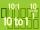Headteacher think whether the distribution of pupils in race in groups of 4,5,6,9 or 10. How many pupils must have at least school at possible options?
• Lcm to eliminate yIdentify the least common multiple that would eliminate the y-variable. 6x - 5y = -4 4x + 2y = 28
• Lesson exercising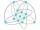The lesson of physical education, pupils are first divided into three groups so that each has the same number. The they redistributed, but into six groups. And again, it was the same number of children in each group. Finally they divided into nine equal g
• Lcm of three numbersWhat is the Lcm of 120 15 and 5
• Dining tables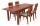In the dining room are tables with 4 chairs, 6 chairs, 8 chairs. How many diners must be at least to occupy all tables (chairs) and diners are more than 50?
• Plum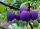On the platter are plum. How many were there if its have to be able to share equally among 8,10 and 12 children?
• 3 buses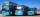At morning 5:00 am three buses started from one place. The first travel in five-minute intervals, the second at 10-minute intervals and the third at 25-minute intervals. At what hour will again be the three buses coming from the same place?
• Summer camp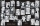Some boys or girls signed up for the summer camp, which has a maximum capacity of 200 children. The main leader noticed that during the evening start, he could arrange the participants exactly in the twelve-step, sixteen-step, or eighteen-step, and no one